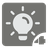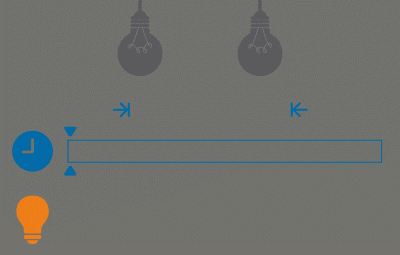Multiple Lightbulbs
Simple+
English RU

In the 4th mission of the series more light bulbs are added.

You still must determine how long the room will be lit during the observation period between start_watching and end_watching. But now we have more than one light bulb. This means that in the light bulb switching array can now also be passed the number of the light bulb, the button of which is being pressed.

Each element of the button clicking array can be either a datetime object (which means the time when the first button was pressed) or a tuple of 2 elements (where the first elements is a datetime object, the time the button was pressed), and the second is the number of the light bulb, the button of which is being pressed.

If the passed array will only consist of datetime elements, then we have only one light bulb and the function should work the same way as in the previous mission of the series.Input: Three arguments and only the first one is required. The first one is a list of datetime objects (instead of datetime object there can be a tuple of two datetime and int), the second and the third ones are the datetime objects.

Output: A number of seconds as an integer.

Example:

```sum_light([
datetime(2015, 1, 12, 10, 0, 0),
datetime(2015, 1, 12, 10, 0, 10),
(datetime(2015, 1, 12, 10, 0, 0), 2),
(datetime(2015, 1, 12, 10, 1, 0), 2),
]) == 60

sum_light([
(datetime(2015, 1, 12, 10, 0, 10), 3),
datetime(2015, 1, 12, 10, 0, 20),
(datetime(2015, 1, 12, 10, 0, 30), 3),
(datetime(2015, 1, 12, 10, 0, 30), 2),
datetime(2015, 1, 12, 10, 0, 40),
(datetime(2015, 1, 12, 10, 0, 50), 2),
(datetime(2015, 1, 12, 10, 1, 0), 3),
(datetime(2015, 1, 12, 10, 1, 20), 3),
]) == 60

sum_light([
datetime(2015, 1, 12, 10, 0, 20),
(datetime(2015, 1, 12, 10, 0, 30), 2),
datetime(2015, 1, 12, 10, 0, 40),
(datetime(2015, 1, 12, 10, 0, 50), 2),
], datetime(2015, 1, 12, 10, 0, 30)) == 20

sum_light([
datetime(2015, 1, 12, 10, 0, 0),
datetime(2015, 1, 12, 10, 0, 10),
(datetime(2015, 1, 12, 10, 0, 0), 2),
(datetime(2015, 1, 12, 10, 1, 0), 2),
], datetime(2015, 1, 12, 10, 0, 20), datetime(2015, 1, 12, 10, 1, 0)) == 40
```

Precondition:

• The array of pressing the button is always sorted in ascending order.
• The array of pressing the button has no repeated elements.
• The minimum possible date is 1970-01-01
• The maximum possible date is 9999-12-31